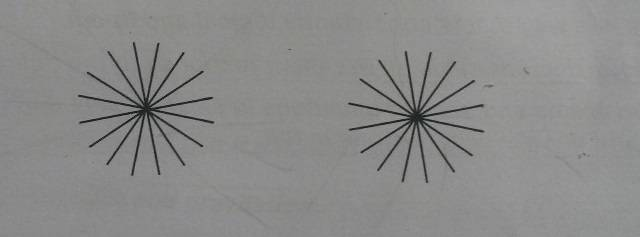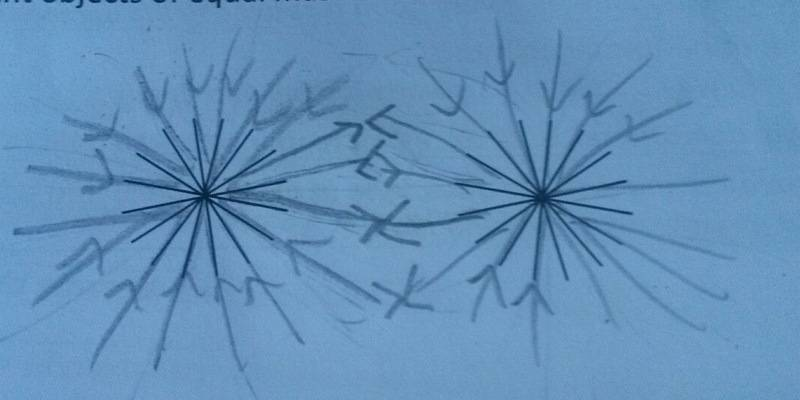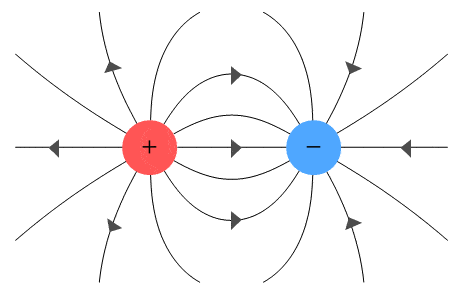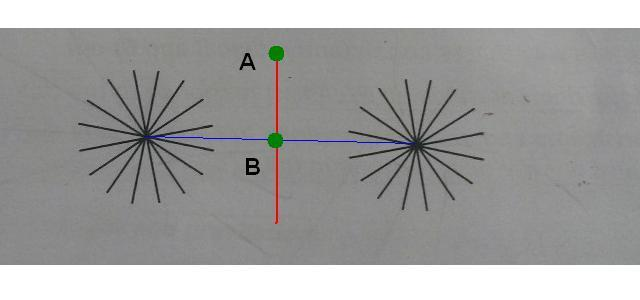# Gravitational Field Lines

## Homework Statement

"Complete the diagram to display gravitational field lines between two objects of equal mass."## Homework Equations

N/A as question is descriptive.

## The Attempt at a Solution

Okay, I know it's a bit of a mess but what I'm trying to show here is that the fields are radial and each mass attracts the other with equal force. Is this correct?If not, please point me in the right direction.

Many thanks!

- Talli

Hm, have you ever seen a picture of electric field-lines between two opposite charges? If there is no outer gravitational field I belive the two masses should yield a similar result. (Coulomb'ss law and Newton's law of gravitation is very similar in structure!). :)

•TalliThePrune
Hm, have you ever seen a picture of electric field-lines between two opposite charges? If there is no outer gravitational field I belive the two masses should yield a similar result. (Coulomb'ss law and Newton's law of gravitation is very similar in structure!). :)

Yeah, I've just been doing questions on that actually!Clearly my sketch isn't the best, I meant it to look similar to this electric field diagram except with arrows pointing at both masses (instead of one way towards the negative/away from the positive). I just can't seem to find any visual representations of two masses online.

SammyS
Staff Emeritus
Homework Helper
Gold Member
Yeah, I've just been doing questions on that actually!Clearly my sketch isn't the best, I meant it to look similar to this electric field diagram except with arrows pointing at both masses (instead of one way towards the negative/away from the positive). I just can't seem to find any visual representations of two masses online.

This is the electric field image you mentioned:Alettix has basically a good idea in that you might consider electric field lines for a case in which point charges in an electric field will behave in a manner analogous to the behavior of point masses in a gravitational field. However, her suggestion to look at the field lines produced by opposite charges may not be what is needed.

This analogy is complicated by the fact that mass has no sign. All mass force is attractive. How do you determine the direction of a force field? Place a test charge (or test mass) at some location and determine the direction of the force exerted on or test charge (or mass) .

We are not particularly interested in whether the two objects producing the field attract each other or repel each other.

Show that the two proposed configurations are not analogous.

How can that be rectified?

•Alettix and berkeman
Imagine that for grater distance the field of two masses must be like as one mass field.

ehild
Homework Helper

## The Attempt at a Solution

Okay, I know it's a bit of a mess but what I'm trying to show here is that the fields are radial and each mass attracts the other with equal force. Is this correct?You placed the arrows nearly correctly, and you are right, the field lines are radial, but only very near to one mass. But you know that the total field is the vector sum of the fields of the individual masses. So what is the direction of the resultant field lines along the symmetry axis (the perpendicular bisector) of the two masses? At A, for example? And what is the net field acting on a small mass at point B?•TalliThePrune